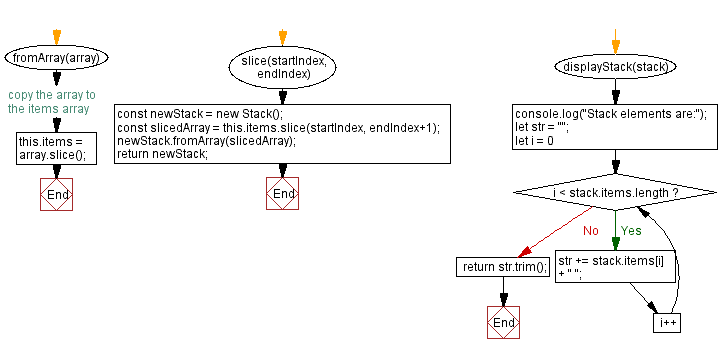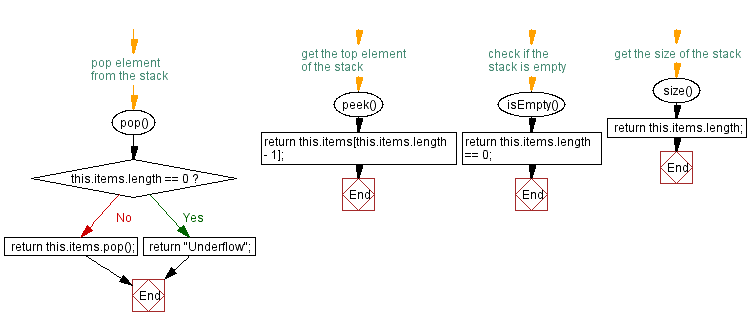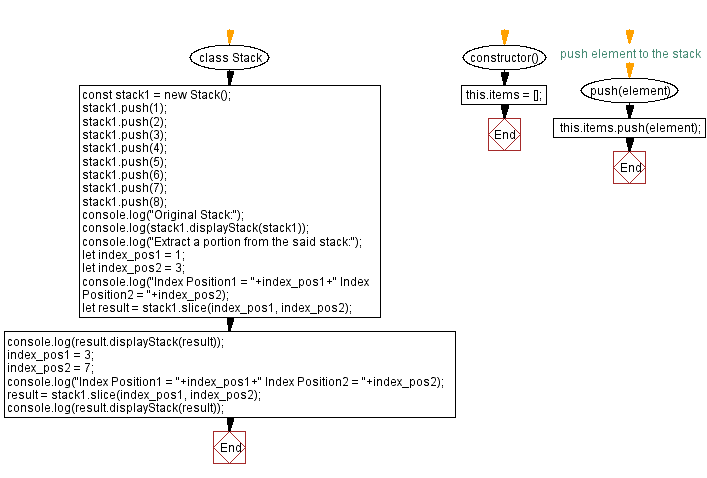# JavaScript Exercises: New stack from a portion of the original stack

## JavaScript Stack: Exercise-32 with Solution

Write a JavaScript program that implements a stack and creates a new stack from a portion of the original stack.

Sample Solution:

JavaScript Code:

``````class Stack {
constructor() {
this.items = [];
}

// push element to the stack
push(element) {
this.items.push(element);
}

// pop element from the stack
pop() {
if (this.items.length == 0) {
return "Underflow";
}
return this.items.pop();
}

// get the top element of the stack
peek() {
return this.items[this.items.length - 1];
}

// check if the stack is empty
isEmpty() {
return this.items.length == 0;
}

// get the size of the stack
size() {
return this.items.length;
}
fromArray(array) {
this.items = array.slice(); // copy the array to the items array
}
slice(startIndex, endIndex) {
const newStack = new Stack();
const slicedArray = this.items.slice(startIndex, endIndex+1);
newStack.fromArray(slicedArray);
return newStack;
}

displayStack(stack) {
console.log("Stack elements are:");
let str = "";
for (let i = 0; i < stack.items.length; i++)
str += stack.items[i] + " ";
return str.trim();
}
}
const stack1 = new Stack();
stack1.push(1);
stack1.push(2);
stack1.push(3);
stack1.push(4);
stack1.push(5);
stack1.push(6);
stack1.push(7);
stack1.push(8);
console.log("Original Stack:");
console.log(stack1.displayStack(stack1));
console.log("Extract a portion from the said stack:");
let index_pos1 = 1;
let index_pos2 = 3;
console.log("Index Position1 = "+index_pos1+" Index Position2 = "+index_pos2);
let result = stack1.slice(index_pos1, index_pos2);
console.log(result.displayStack(result));
index_pos1 = 3;
index_pos2 = 7;
console.log("Index Position1 = "+index_pos1+" Index Position2 = "+index_pos2);
result = stack1.slice(index_pos1, index_pos2);
console.log(result.displayStack(result));
```
```

Sample Output:

```Original Stack:
Stack elements are:
1 2 3 4 5 6 7 8
Extract a portion from the said stack:
Index Position1 = 1 Index Position2 = 3
Stack elements are:
2 3 4
Index Position1 = 3 Index Position2 = 7
Stack elements are:
4 5 6 7 8
```

Flowchart:Live Demo:

See the Pen javascript-stack-exercise-32 by w3resource (@w3resource) on CodePen.

Improve this sample solution and post your code through Disqus

Stack Previous: Symmetric difference of two stacks.
Stack Exercises Next: Verify all stack elements satisfy a condition.

What is the difficulty level of this exercise?

Test your Programming skills with w3resource's quiz.

﻿